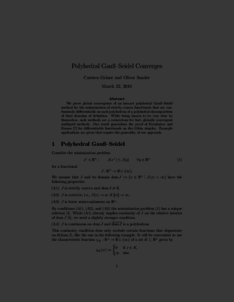Repository: Freie Universität Berlin, Math Department

# Polyhedral Gauß-Seidel converges

Gräser, C. and Sander, O. (2014) Polyhedral Gauß-Seidel converges. Journal of Numerical Mathematics, 22 (3). pp. 221-254. ISSN 1570-2820Preview

164kB

Official URL: http://dx.doi.org/10.1515/jnma-2014-0010

## Abstract

We prove global convergence of an inexact extended polyhedral Gauß-Seidel method for the minimization of strictly convex functionals that are continuously differentiable on each polyhedron of a polyhedral decomposition of their domains of definition. While pure Gauß-Seidel methods are known to be very slow for problems governed by partial differential equations, the presented convergence result also covers multilevel methods that extend the Gauß-Seidel step by coarse level corrections. Our result generalizes the proof of  for differentiable functionals on the Gibbs simplex. Example applications are given that require the generality of our approach.

Item Type: Article Allen-Cahn equation, discontinuous Galerkin methods, global convergence, multigrid methods, polyhedral Gauß-Seidel Mathematical and Computer Sciences > Mathematics > Numerical Analysis Department of Mathematics and Computer Science > Institute of Mathematics 1789 Ekaterina Engel 17 Feb 2016 09:49 03 Mar 2017 14:41

Repository Staff Only: item control page